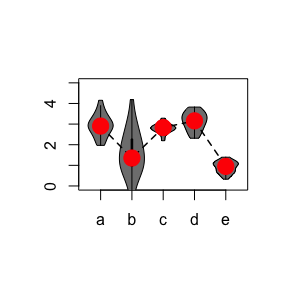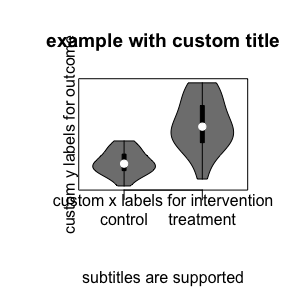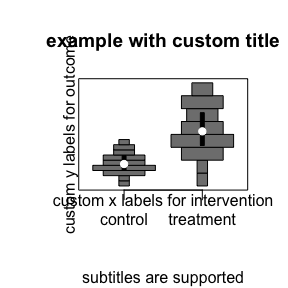# Overlaying base R graphics

## Introduction: Integration with base R graphics

Here we demonstrate how to combine violin plots with other base R graphics. In principle any base R graphics can be overlayed on top of a violin plot for annotation.

Many problems can be resolved by overlaying base R graphics and integrating vioplot with other plotting functions. Any additional elements can be overlayed by running commands after generating the plot. The x-axes are integer values [1,2,3,…] for each violin. The y-axes are continuous values as displayed.

The following plotting elements are supported for example: points, lines, polygon

It is also possible to modify plotting parameters with: title, axis, legend

“vioplot()” functions similar to “plot()” and passes input arguments from “par()”.

### Plotting violins with highlighted medians

# generate dummy data
a <- rnorm(25, 3, 0.5)
b <- rnorm(25, 2, 1.0)
c <- rnorm(25, 2.75, 0.25)
d <- rnorm(25, 3.15, 0.375)
e <- rnorm(25, 1, 0.25)
datamat <- cbind(a, b, c, d, e)
dim(datamat)
##  25  5
library("vioplot")
vioplot(datamat, ylim = c(0, 5))
# compute medians
data.med <- apply(datamat, 2, median)
data.med
##         a         b         c         d         e
## 2.9040581 1.3545198 2.8318172 3.1564032 0.9491974
#overlay medians
lines(data.med, lty = 2, lwd = 1.5)
points(data.med, pch = 19, col = "red", cex = 2.25)### Custom axes and titles

It is also possible to modify the axes labels and titles as shown in this example. Here default axes are suppressed and replaced with custom parameters.

outcome <- c(rnorm(25, 3, 1), rnorm(25, 2, 0.5))
intervention <- c(rep("treatment", 25), rep("control", 25))
table(intervention)
## intervention
##   control treatment
##        25        25
names(table(intervention))
##  "control"   "treatment"
unique(sort(intervention))
##  "control"   "treatment"
intervention <- as.factor(intervention)
levels(intervention)
##  "control"   "treatment"
d <- data.frame(outcome, intervention)
vioplot(outcome ~ intervention, data = d, xaxt = 'n', yaxt = 'n',
main = "", xlab = "", ylab = "")
axis(side = 1, at = 1:length(levels(intervention)), labels = levels(intervention))
mtext("custom x labels for intervention", side = 1)
mtext("custom y labels for outcome", side = 2)
title(main = "example with custom title", sub = "subtitles are supported")#### Annotated histograms

This is also supported by the histogram plot.

histoplot(outcome ~ intervention, data = d, xaxt = 'n', yaxt = 'n',
main = "", xlab = "", ylab = "")
axis(side = 1, at = 1:length(levels(intervention)), labels = levels(intervention))
mtext("custom x labels for intervention", side = 1)
mtext("custom y labels for outcome", side = 2)
title(main = "example with custom title", sub = "subtitles are supported")